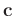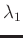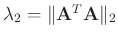# Appendix: Local similarity

Local similarity between vectorsandis defined as:(10)

wheredenotes element-wise product.andcome from two least-squares minimization problems:(11)(12)

Here,is a diagonal operator composed of the elements of,is a diagonal operator composed of the elements of. Note that in equations 10-12,,, anddenote vectorized 2D matrices. Equations 11 and 12 can be solved using shaping regularization with a local-smoothness constraint:(13)(14)

whereis a smoothing operator,andare two parameters controlling the physical dimensionality and enabling fast convergence when inversions forandexpressed in equations 13 and 14 are implemented iteratively.andcan be chosen asand.

2020-02-10